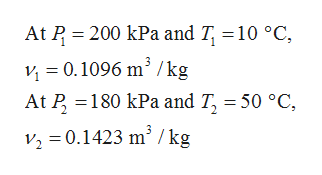# Refrigerant R-134a enters a 30cm diameter pipe (circular cross-section) at an absolute pressure of 200 kPa and a temperature of 10 °C with a velocity of 5 m/sec. Heat is transferred to the refrigerant as it flows and the gas leaves the pipe at 180 kPa and 50 °C. Find the following quantities:a. The volume flow rate at the exit in m3 /sec.b. The exit velocity in m/sec.

Question
15 views

Refrigerant R-134a enters a 30cm diameter pipe (circular cross-section) at an absolute pressure of 200 kPa and a temperature of 10 °C with a velocity of 5 m/sec. Heat is transferred to the refrigerant as it flows and the gas leaves the pipe at 180 kPa and 50 °C. Find the following quantities:

a. The volume flow rate at the exit in m3 /sec.
b. The exit velocity in m/sec.

check_circle

Step 1

Given data:

The diameter of the pipe, d = 30 cm.

The absolute pressure at inlet, P1 = 200 kPa.

The absolute pressure at inlet, P2 = 180 kPa.

The inlet temperature, T1= 10 oC.

The exit temperature, T2 = 50 oC.

The velocity at the inlet, v1 = 5 m/s.

Step 2

From the property table of refrigerant R-134a, obtain the value of specific volume at the inlet and the outlet of the pipe.help_outlineImage TranscriptioncloseAt P 200 kPa and T, 10 °C v 0.1096 m2/kg At P 180 kPa and T, 50 °C v3 = 0.1423 m3 / kg fullscreen
Step 3

Calculate the volumetric flo...

### Want to see the full answer?

See Solution

#### Want to see this answer and more?

Solutions are written by subject experts who are available 24/7. Questions are typically answered within 1 hour.*

See Solution
*Response times may vary by subject and question.
Tagged in

### Mechanical Engineering